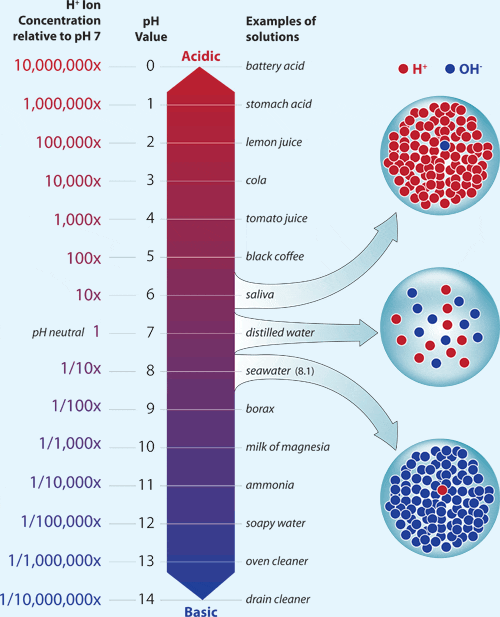It looks like you're using Internet Explorer 11 or older. This website works best with modern browsers such as the latest versions of Chrome, Firefox, Safari, and Edge. If you continue with this browser, you may see unexpected results.

# Exponential and Logarithmic Functions

## Introduction

There are various real-life applications that can be modelled using exponential/logarithmic functions.

 For exponential functions, these include: population growth compound interest isotope halflife. For logarithmic functions, these include: hydrogen ion concentration (pH scale) earthquake intensity (Richter scale) sound intensity (decibel scale).

We will describe a few of these applications and work through some related word-problem examples in the following sections.## Exponential Growth/Decay

Data such as population can be modeled using exponential growth/decay. The general formula used in modeling and calculations involving exponential growth/decay is:

$$A=A_0b^t$$,

where:

• $$A$$ is the total value/amount,
• $$A_0$$ is the initial value/amount,
• $$b$$ is the factor of growth/decay (calculated as $$b=1+r$$ for growth rate $$r$$ or $$b=1-r$$ for decay rate $$r$$),
• $$t$$ is the number of time periods

We will use this formula in the following example:

Example: The population of Apple County in 2015 was estimated to be 45,000 people with an annual rate of increase of 2.5%.
 a) What is the growth factor for the population of Apple County? b) Write an equation that models the population growth in Apple County. c) Use the equation from part b) to estimate the population of Apple County in 2025 (rounded to the nearest whole number).

Solution:

a) Since the rate of growth is $$r=2.5\%=0.025$$, the growth factor is $$b=1+r=1+0.025=$$$$1.025$$.

b) From the information given in the question, we have that $$A_0=45000$$, and that the growth factor is $$b=1.025$$ (from part a)). Substituting these values into the general formula gives:

$$A=45000(1.025)^t$$, where $$t$$ is the number of years after 2015

c) Since 2025 is 10 years after 2015, $$t=10$$. Substituting this value into the equation we found in part b) gives the estimate for the population of Apple County in 2025:

$$A=45000(1.025)^{10}= \$$$$57604$$

## Isotope Halflife

Many radioactive isotopic substances' masses decrease over time, and a useful piece of information when working with such substances in chemistry is their half-life - the amount of time required for an amount to decrease to half its initial quantity. A general formula used when doing calculations involving half-life is:

$$M=A(\frac{1}{2})^{\frac{t}{h}}$$

where:

• $$M$$ is the remaining or current mass/amount,
• $$A$$ is the initial amount/mass,
• $$t$$ is the time elapsed,
• $$h$$ is the half-life.

We will use this formula in the following example:

Example: Chris works in a laboratory and uses radioactive substances. He received a shipment of 350g of radioactive radium, then 2 weeks later, 23.5g of the radium remained. What is the half-life of radium (rounded to two decimal places)?

Solution:

From the information given in the question, we have that $$A=350, M=23.5, t=14 (days)$$. So substituting these values into the general formula and solving for the half-life $$h$$ gives:

$$23.5=350(\frac{1}{2})^{\frac{14}{h}}$$

$$\frac{23.5}{350}=(\frac{1}{2})^{\frac{14}{h}}$$

$$\frac{47}{700}=(\frac{1}{2})^{\frac{14}{h}}$$

$$\frac{14}{h}=log_{\frac{1}{2}}(\frac{47}{700})=\frac{log(\frac{47}{700})}{log(\frac{1}{2})} \approx 3.90$$

$$h=\frac{14}{3.90}=$$$$\ 3.59$$

So, the half-life of radium is 3.59 days (this means that after 3.59 days, there will only remain half of the original amount of radium).

## pH and Hydrogen Ion Concentration

The pH scale, often used in chemistry, measures a substance's acidity or alkalinity by giving it a pH value of 0 to 14. The concentration of hydrogen ions (compared to a substance with pH of 7) in a substance is directly related to its pH value, specifically, it follows the below relationship/equation:

$$pH=-log[H^+]$$,

where:

• $$pH$$ is the pH value (number between 0-14),
• $$[H^+]$$ is the concentration of hydrogen ions (measured in mol/L).

Note: Every difference of one between pH values corresponds to a tenfold (or 10 times) change in the concentration of hydrogen ions in a substance, hence the use of a logarithmic scale (of base 10).We will use this formula in the following examples:

Example: Calculate the pH value if the concentration of hydrogen ions is 0.00002 mol/L.

Solution: We're given that $$[H^+]=0.00002$$, so substituting this value into the $$pH$$-$$[H^+]$$ equation gives the pH value of:

$$pH=-log(0.00002) \approx$$$$4.7$$

Example: The pH of tomato juice is 4. Calculate its hydrogen ion concentration in mol/L.

Solution: We're given that pH = 4, so substituting this value into the $$pH$$-$$[H^+]$$ equation and solving for $$[H^+]$$ gives:

$$4=-log[H^+]$$

$$-4=log[H^+]$$

$$[H^+]=10^{-4}=$$$$0.0001 mol/L$$

Example: How much stronger is an acid with pH 1.4 than an acid with pH 2.6 (rounded to two decimal places)? (Note that hydrogen ion concentration is a measure of the strength of an acid).

Solution: We first find the hydrogen ion concentrations of both acids by using the $$pH$$-$$[H^+]$$ equation, then use their pH values to compare their acidity strength.

 Acid with pH 1.4 Acid with pH 2.6 $$1.4=-log[H^+]$$ $$-1.4=log[H^+]$$ $$[H^+]=10^{-1.4} \approx 0.03981$$ $$2.6=-log[H^+]$$ $$-2.6=log[H^+]$$ $$[H^+]=10^{-2.6} \approx 0.00251$$

To compare the strength of the first acid with the second, we divide the hydrogen ion concentration of the first acid by the concentration of the second:

$$\frac{0.03981}{0.00251} \approx 15.86$$

So, an acid with a pH of 1.4 is approximately $$15.86$$ times stronger than one with pH of 2.6.